# Introduction to AC Circuits

Chapter 2 - Analysis of AC Systems

We started out this chapter by discussing power in DC circuits. Now, we will take a detailed look at alternating current, and we will also discuss power dissipation with respect to AC that is flowing through a purely resistive load.

## What is Alternating Current?

We saw in the first page of this chapter that “AC” refers to current that regularly changes direction or to voltage that regularly changes polarity. AC voltages and currents typically vary according to a sinusoidal pattern: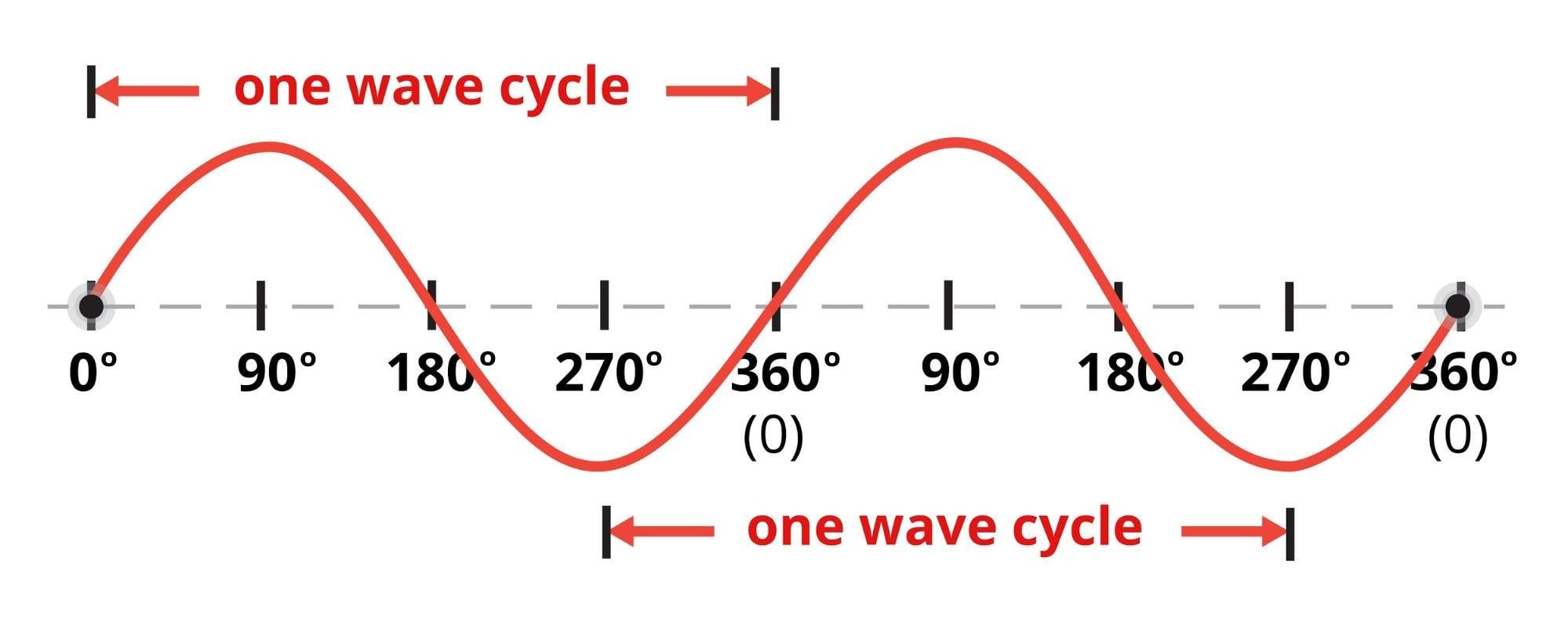Figure 1. A sine wave representing the varying amplitude of an AC voltage or current. At 0° and 180°, the value of a sine wave is zero. At 90° and 270°, it is at its maximum absolute value.

The portion of a sine wave in which the amplitude starts at a given value, reverses polarity, and returns to the initial value is referred to as one cycle. The rate at which a sinusoidal voltage or current changes polarity can be quantified as cycles per second; this corresponds to frequency and is expressed using the unit hertz (abbreviated Hz). In the United States, electrical power is distributed as a sinusoidal voltage that completes 60 cycles every second, i.e., it has a frequency of 60 Hz.

The radian is the SI unit for the measurement of angles. Let’s say that we have a circle of radius r and we draw an arc along the circumference. The angle corresponding to this arc will depend on the length of the arc. If we draw an arc whose length is equal to the radius of the circle, the arc subtends (i.e., forms) an angle of one radian.Figure 2. The radian

To understand the conversion between radians and degrees, note the following: A circle’s circumference is 2πr, and the number of degrees corresponding to the circumference is 360. Thus, if we are thinking in degrees, an arc with a length of 2πr will subtend an angle of 360°, and an arc with a length of r (which is 2πr/2π) will subtend an angle of 360°/2π. Thus, one radian = 360°/2π ≈ 57.3°.

When we’re working with angles in radians, it is often helpful to use angular frequency; the symbol is ω, i.e., lowercase omega (from the Greek alphabet). The unit for normal frequency is cycles per second. One full cycle is equal to 2π radians, which means that a frequency of one cycle per second is equivalent to an angular frequency of 2π radians per second:## Power in AC Circuits

If an AC circuit includes only resistors (i.e., no inductors or capacitors—much more about them later), current and voltage are always in phase, meaning that their amplitudes vary in perfect synchronization. We can directly apply Ohm’s law to circuits such as this: the current through a resistor will vary according to the sinusoidal shape of the voltage, but the instantaneous value of the current will always be equal to the voltage across the resistor divided by the resistance.

If we apply an AC voltage to a purely resistive load, the resistor’s voltage and current with respect to time can be expressed as follows: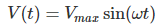The following plot depicts sinusoidal current through and voltage across a resistor.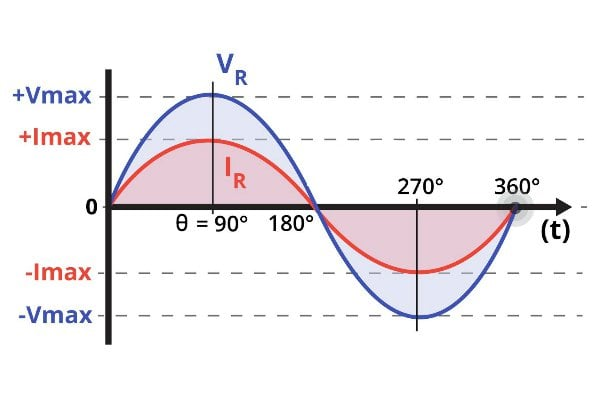Figure 3. Sinusoidal voltage and current in a resistive load.

The expression for the instantaneous power dissipated by a resistor in an AC circuit is obtained as follows: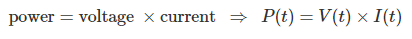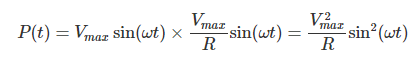## RMS Voltage

Let’s say you have a circuit consisting of a 12 V sinusoidal supply voltage and a resistive load. When we say “twelve volts” in this context, we are referring to the maximum amplitude. Thus, if you use 12 V when calculating power dissipation, the result will be the maximum instantaneous power dissipation.

If you look at the sine wave in Figure 3, you can readily see that a large portion of the waveform has an absolute value that is far below the maximum. This means that the average power dissipation will be significantly lower than the maximum power dissipation. What we need is a way to compare an AC voltage to an equivalent DC voltage—equivalent in the sense that the average power dissipation created by the AC signal is equal to the power dissipation of the corresponding DC value.

If we recall that power is proportional to voltage squared, it is not surprising that average-power calculations involving AC signals make use of the root mean square (abbreviated RMS). As the name implies, this quantity is produced by finding the square root of the average of the squared values of a given signal.

The RMS value of a sinusoidal AC signal is calculated as follows: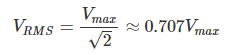The mains voltage in the United States is approximately 115 VRMS, and that means that the maximum value of a typical household AC voltage supply is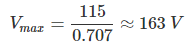## Power in AC Circuits Review

We’ve introduced some basic concepts related to power dissipation in circuits that have sinusoidal voltages and currents.

In the next page, we’ll learn about two fundamental components that are used in countless electrical systems and electronic devices.